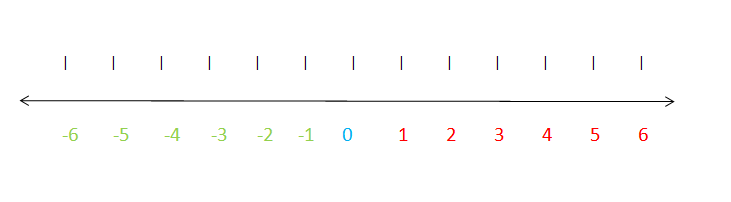## Introduction

In this lesson, we will review how to add integers. Remember that integers are numbers that are distributed into 2 categories: positives and negatives. Integers don't include of any fractions so they are whole numbers. Also, the number 0 (zero) is neither a positive or a negative number.Figure 1 Addition of Integers Number Line

Let's say that we have to add the following numbers:

4 + -9

The number 4 does not have a sign in front of it. If a number doesn't have a sign, it means it is a positive number.

Now we have positive 4 and negative 9.

Let's look at it like this:

We have 4 dollars (+4) and we owe 9 dollars (-9) to a friend. In order to add this we first look at the sign of the larger number.

We know that 9 is greater than 4 so our answer should equal a negative number since 9 has the negative sign in front of it.

Also, since 9 is the greater number, we will use it's operation. 9 is a negative and we know that negative means subtraction. So, if we subtract 9-4 we get 5.
Remember that our answer must be a negative. So we have -5.

That means that if we have \$4 an owe owe \$9 to a friend, we still owe them \$5.

4 + -9 = -5

#### 1 comment:

1.hfeffjhr,jfdjmfjkvcnm